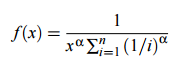# Zeta Distribution (Zipf Distribution)

Probability Distributions > Zeta Distribution (Zipf Distribution)

## What is the Zeta Distribution?

The Zeta Distribution (also called the Zipf distribution, after American linguist George Zipf) is a member of the family of general exponential distributions, used to model the size or ranks of randomly chosen objects from certain population types. In general, it’s used to show the relative popularity of a small subset of a population.

The zeta distribution comes from Zipf’s law, which states that, given a list of the most frequent words in an arbitrary book, the most frequent word will appear twice as often as the second most frequent word, which will appear twice as often as the third most frequent, and so on. In its simplest form, Zipf’s law is the same as the power law.

It can also be used to model a variety of phenomena where there is a large step down from the top (or bottom) to the next level. For example:

• Corporation sizes; large conglomerates tend to be many times larger than their immediate neighbors,
• Income rankings; the top 1% holds most of the wealth, with significant drops down to the 99%,
• Library books: a handful of bestsellers tend to be the most popular in a library.

The probability density function for the zeta distribution is:Where:

X ∼ Zipf(α,n) indicates the random variable X has a Zeta distribution with parameters α and n.

This distribution is also called:

• Discrete Pareto Distribution,
• Joos Model,
• Riemann Zeta Distr.,
• Zipf-Estoup Law,*
• Zipf law.*

*Technically not correct as the distribution was derived from these laws; these laws themselves result in the distribution but are not the actual distributions.

References
Johnson, N. and Kotz, S. (1969) Discrete Distributions. Houghton Mifflin.
Johnson N. L., Kotz S., and Balakrishnan N. (1993) Univariate Discrete Distributions, 2nd ed. New York: Wiley.

CITE THIS AS:
Stephanie Glen. "Zeta Distribution (Zipf Distribution)" From StatisticsHowTo.com: Elementary Statistics for the rest of us! https://www.statisticshowto.com/zeta-distribution-zipf/